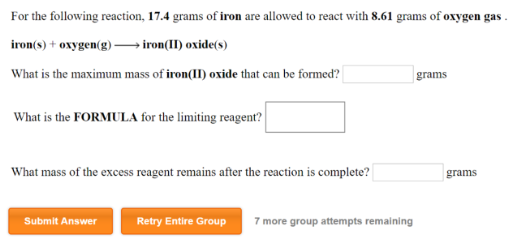# Problem: For the following reaction, 17.4 grams of iron are allowed to react with 8.61 grams of oxygen gas.iron (s) + oxygen (g) → iron (II) oxide(s) What is the maximum mass of iron(II) oxide that can be formed? What is the FORMULA for the limiting reagent? What mass of the excess reagent remains after the reaction is complete?

###### FREE Expert Solution
89% (4 ratings)###### Problem Details

For the following reaction, 17.4 grams of iron are allowed to react with 8.61 grams of oxygen gas.

iron (s) + oxygen (g) → iron (II) oxide(s)

What is the maximum mass of iron(II) oxide that can be formed?

What is the FORMULA for the limiting reagent?

What mass of the excess reagent remains after the reaction is complete?